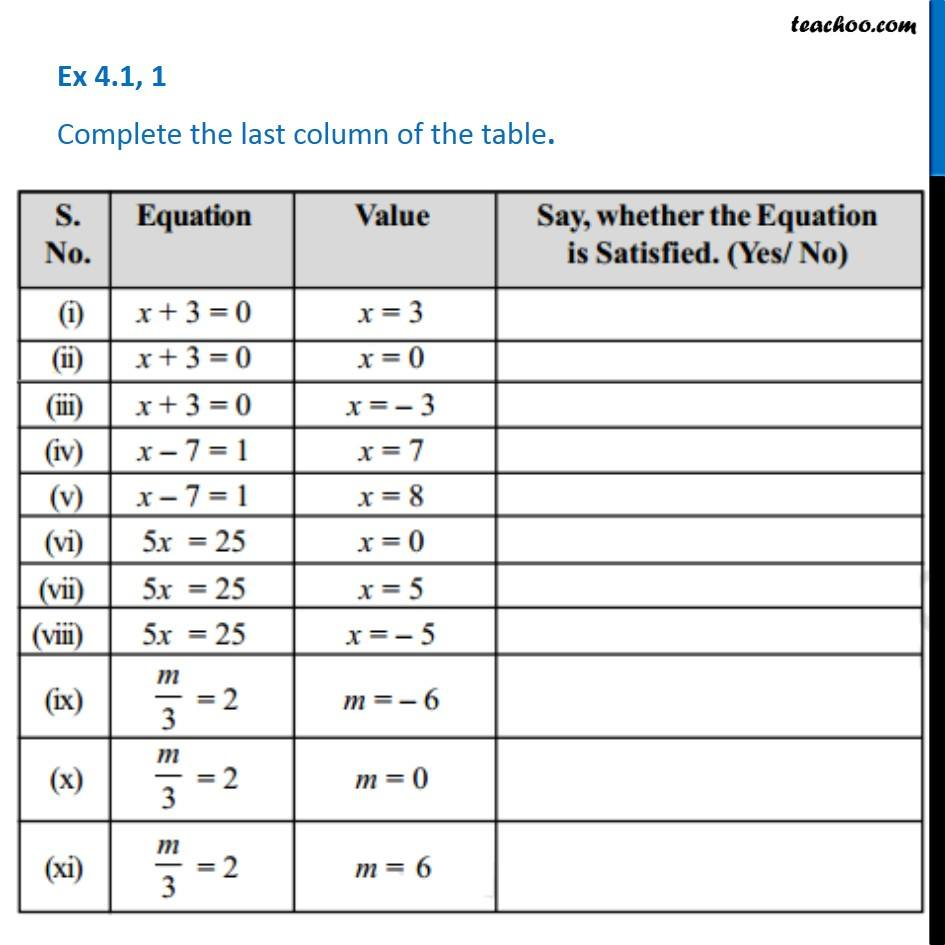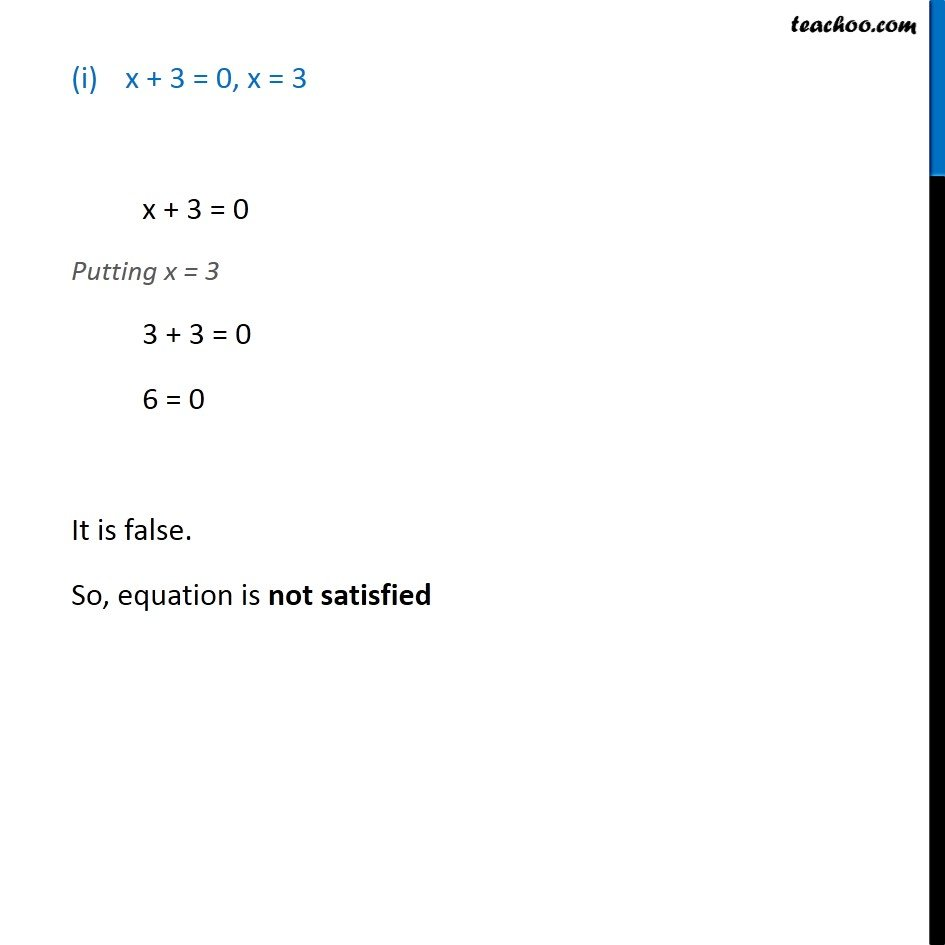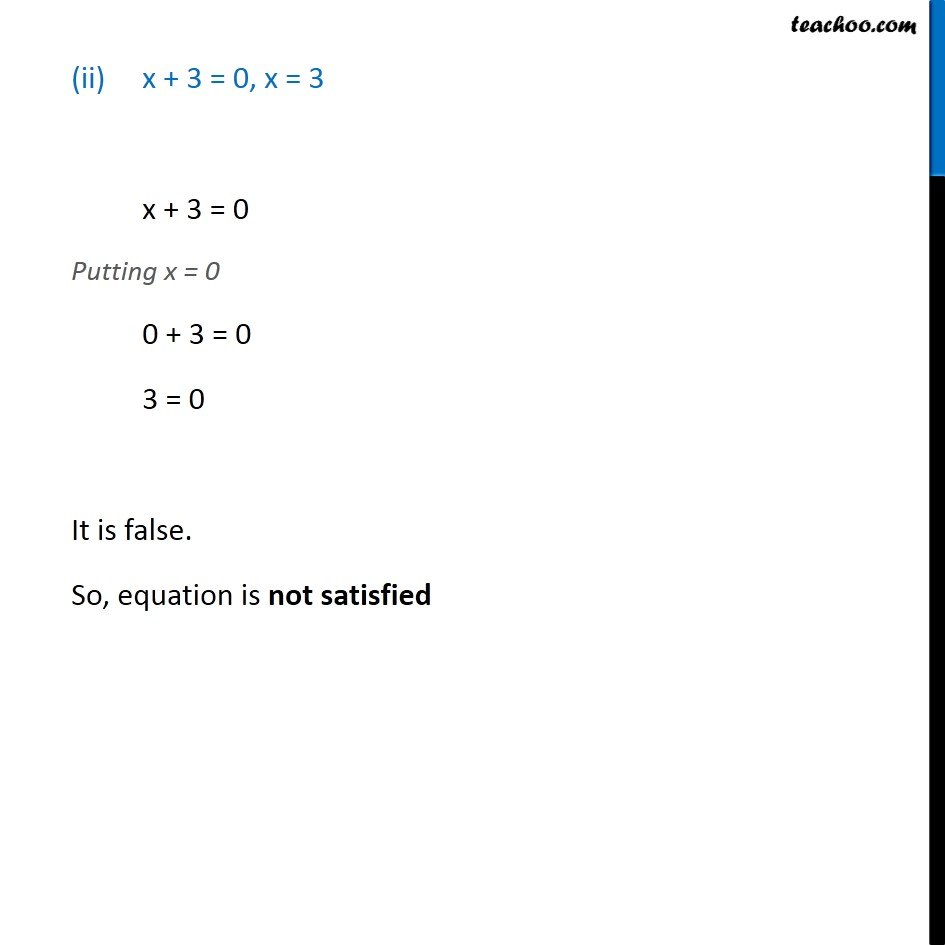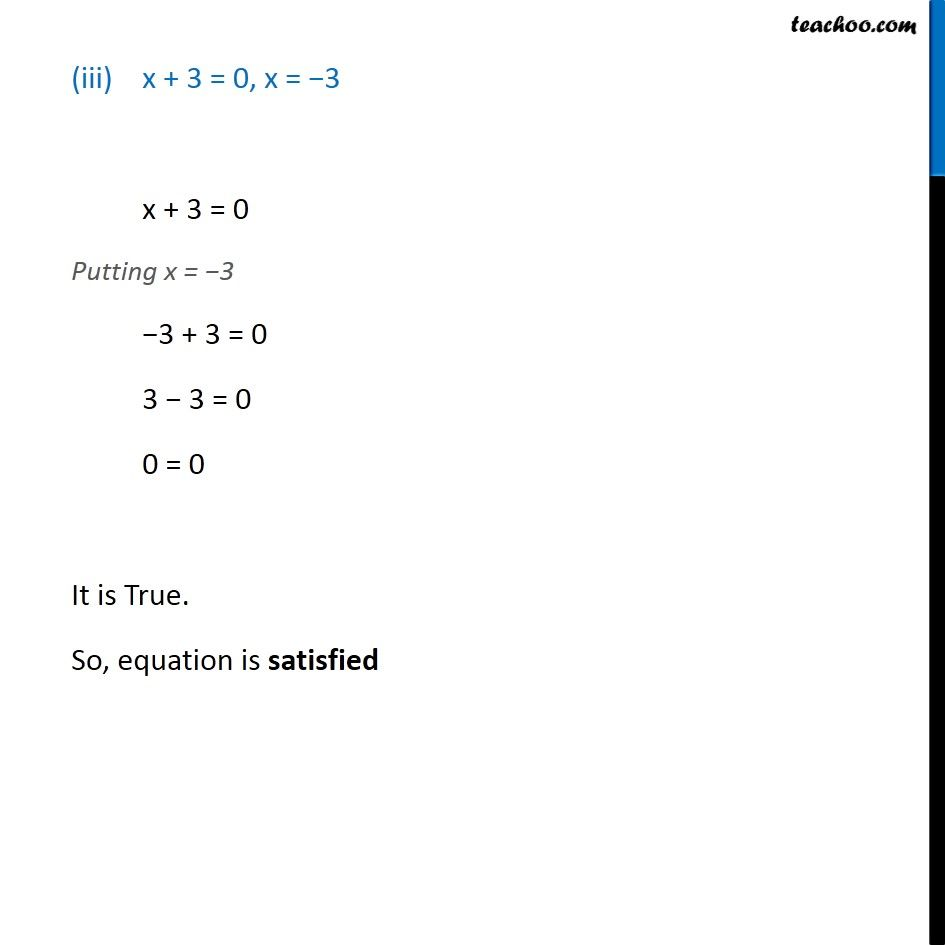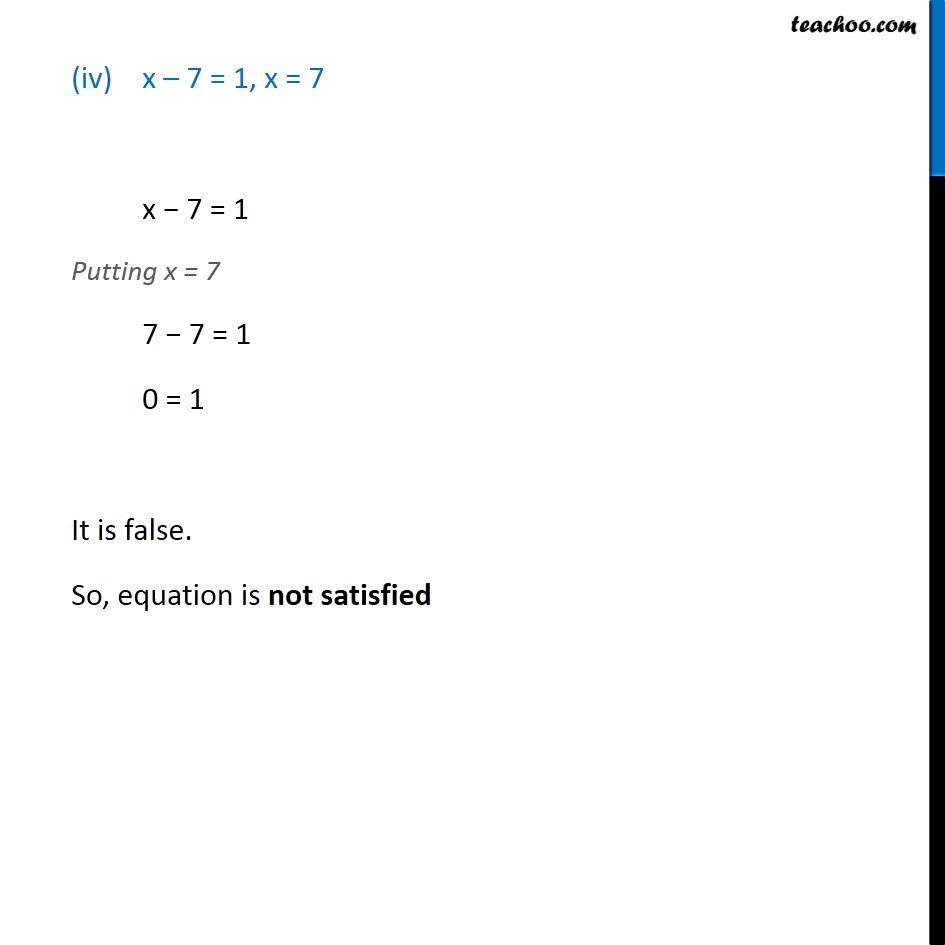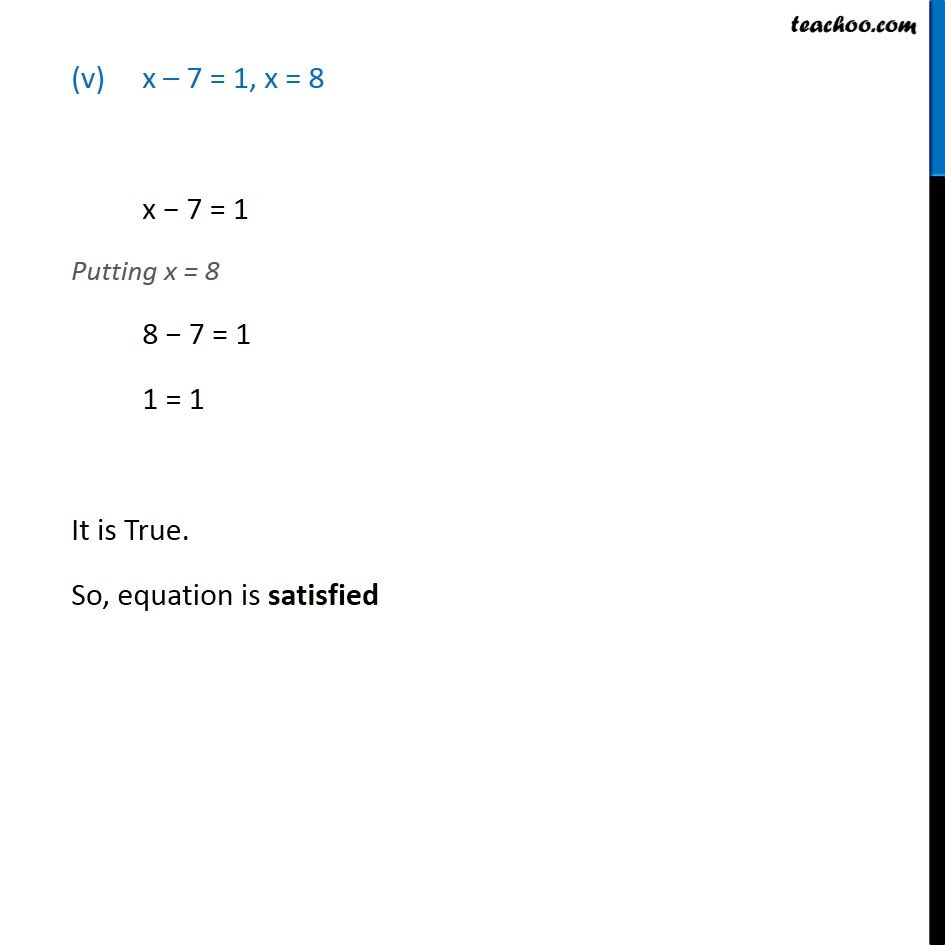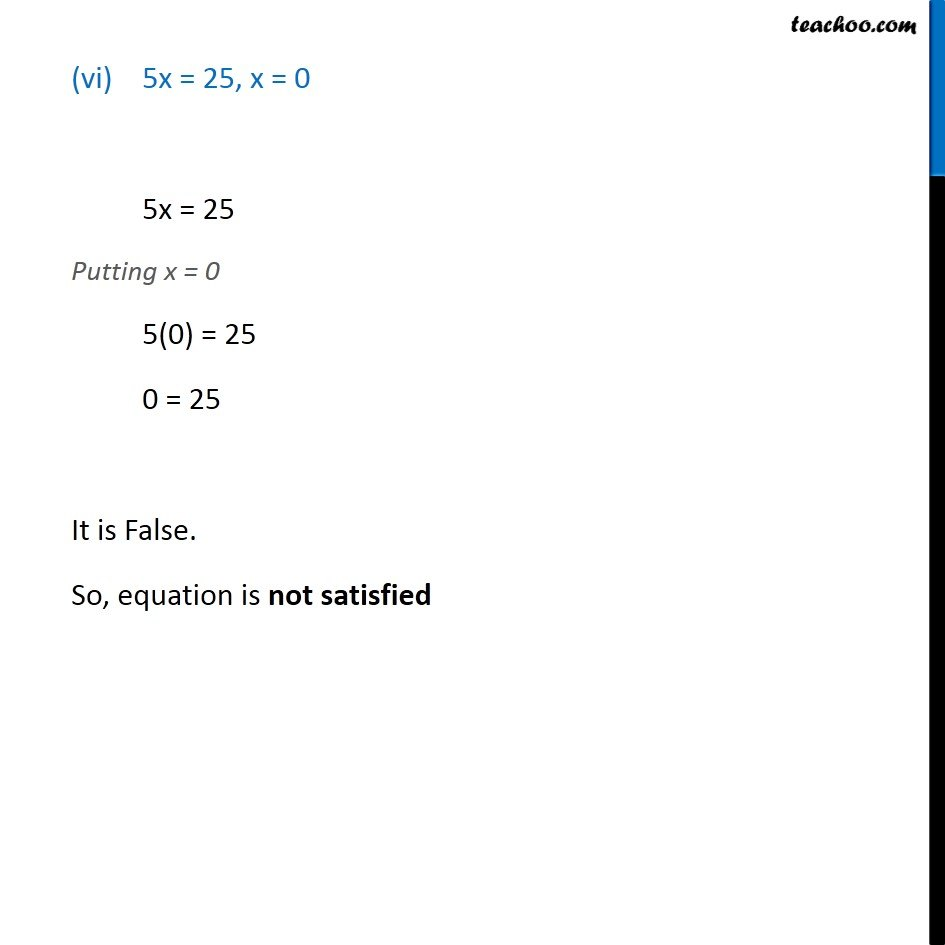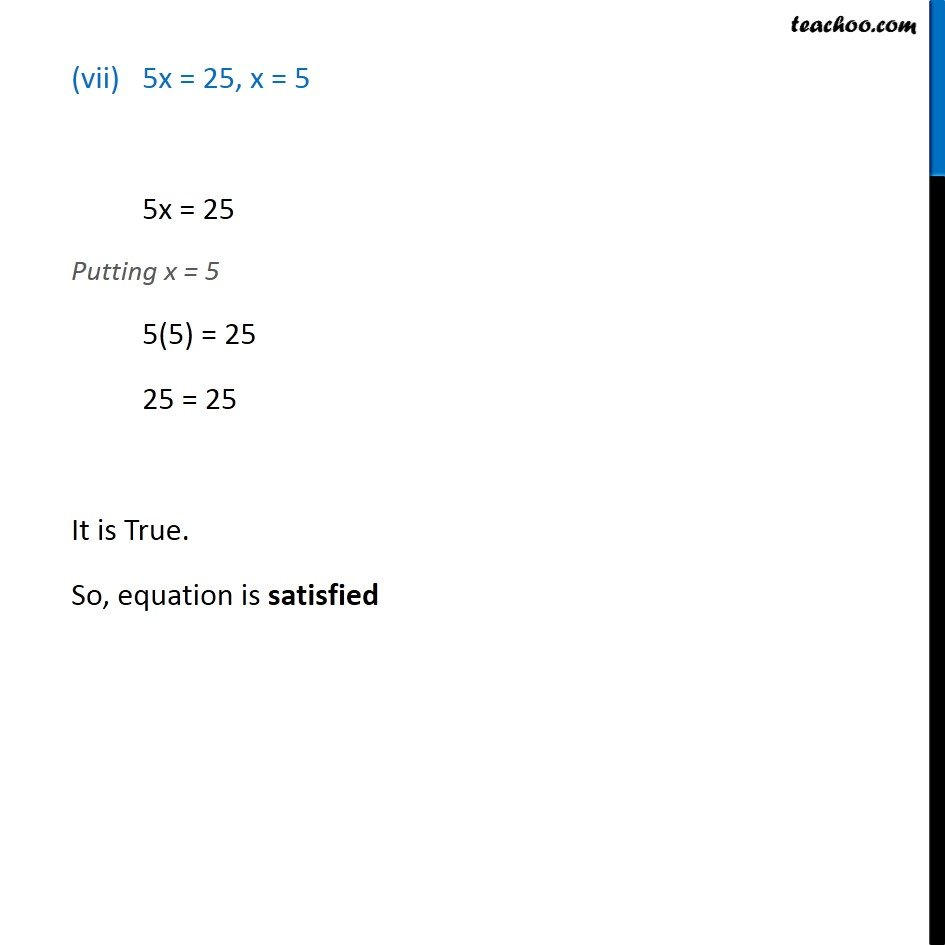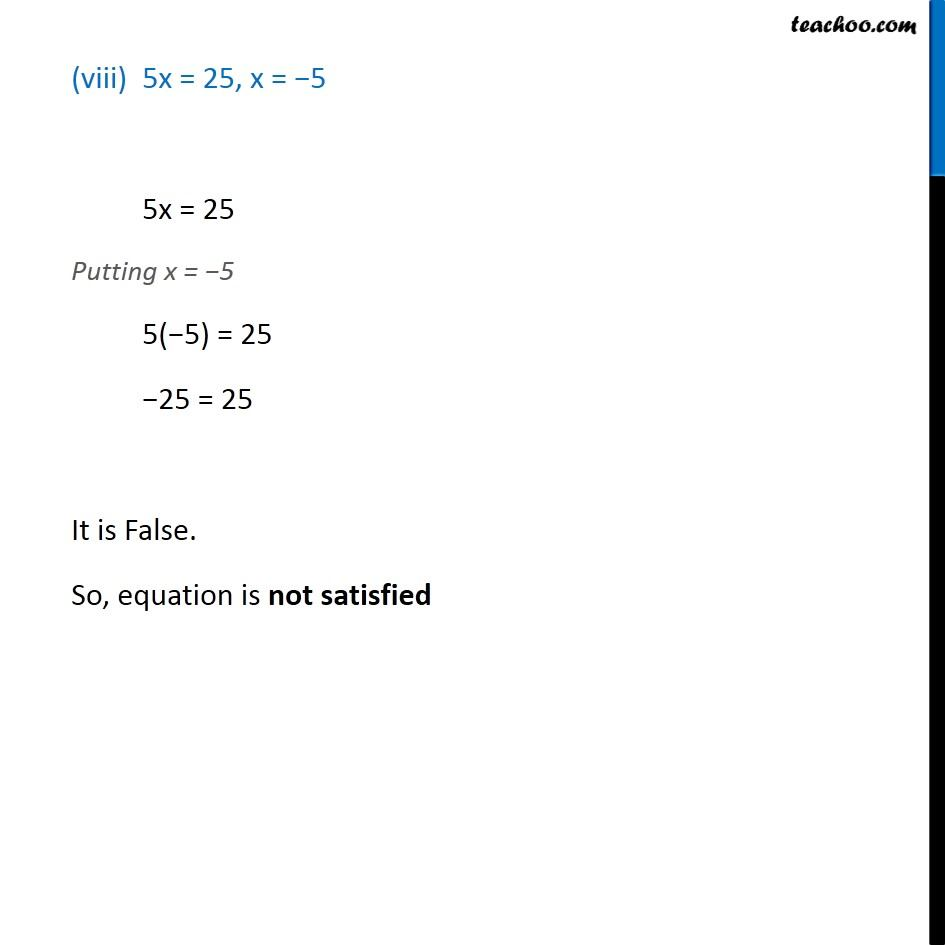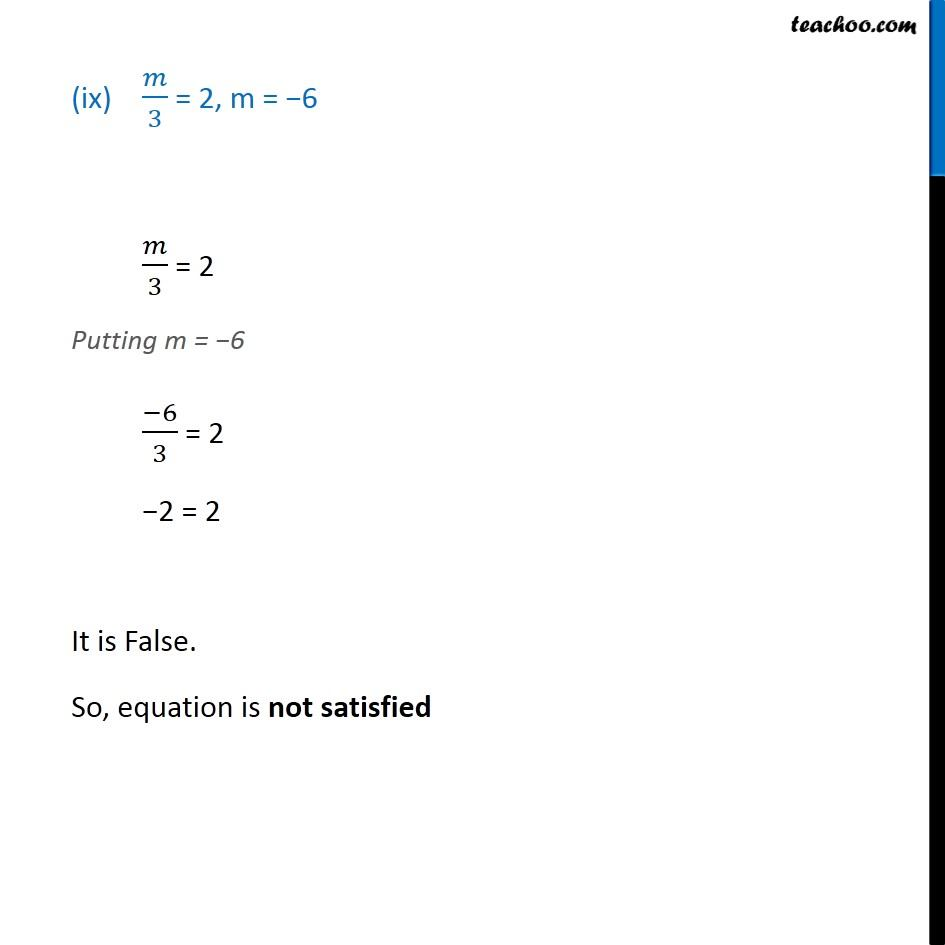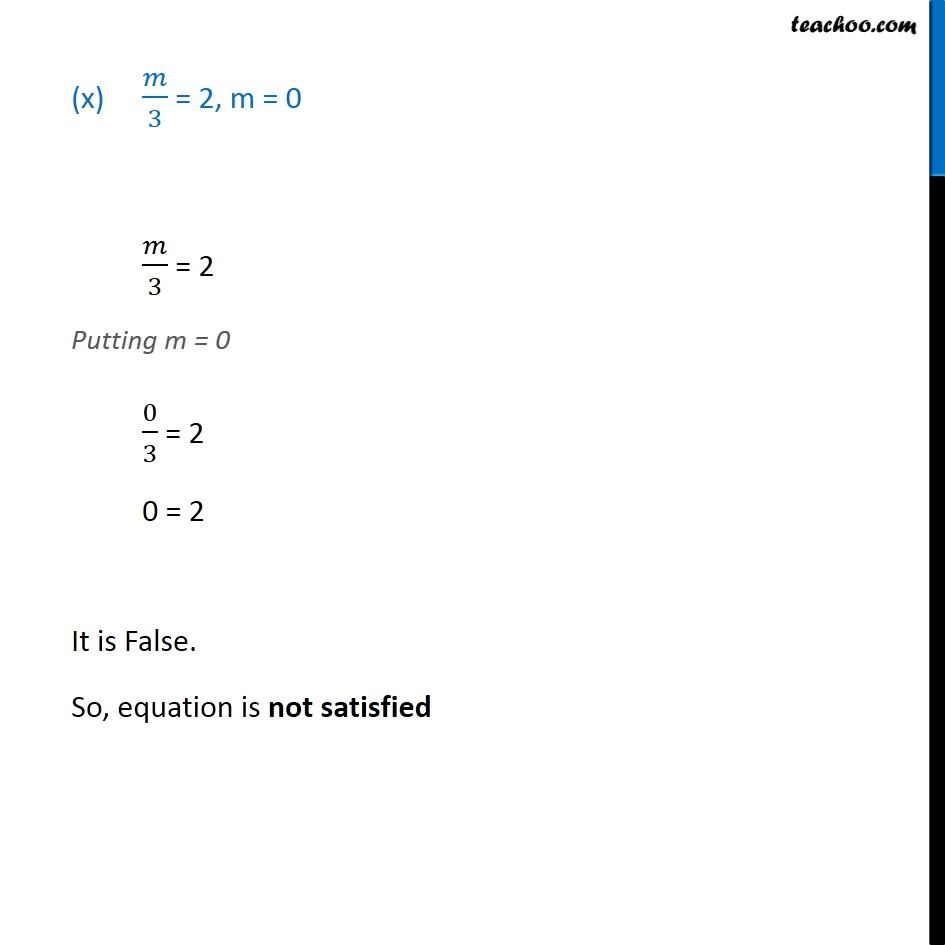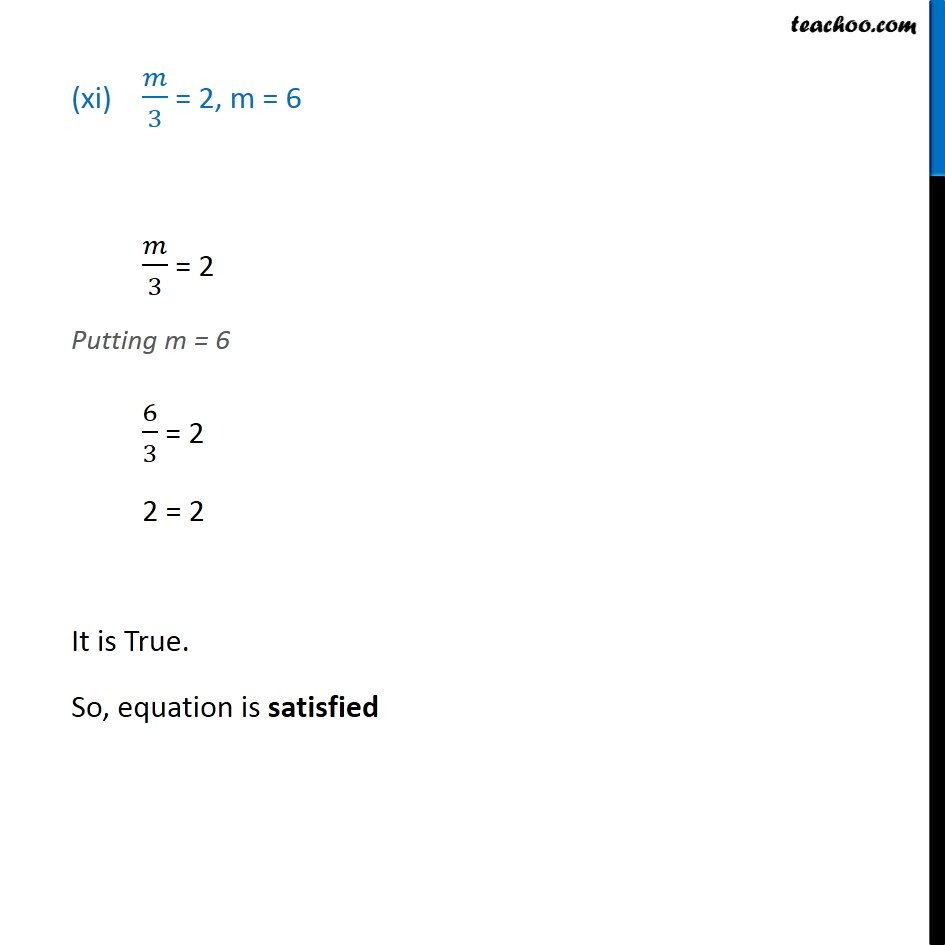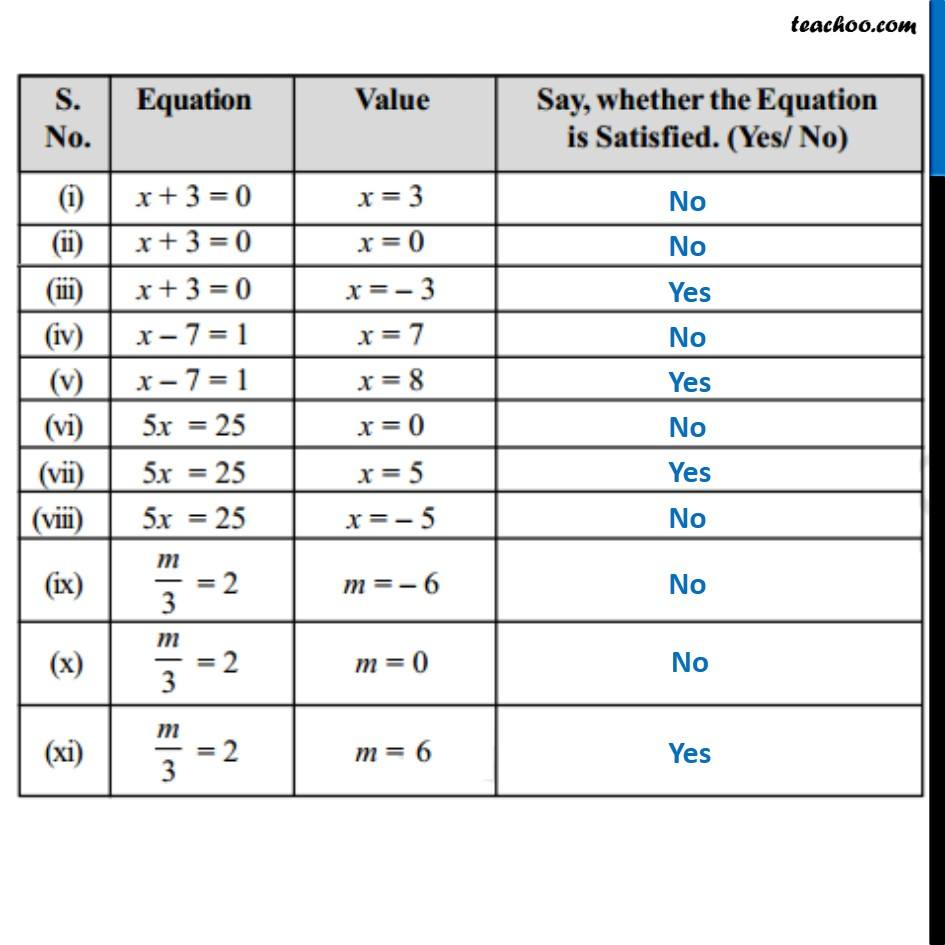1. Chapter 4 Class 7 Simple Equations
2. Concept wise
3. Definition

Transcript

x + 3 = 0, x = 3 x + 3 = 0 Putting x = 3 3 + 3 = 0 6 = 0 It is false. So, equation is not satisfied (ii) x + 3 = 0, x = 3 x + 3 = 0 Putting x = 0 0 + 3 = 0 3 = 0 It is false. So, equation is not satisfied (ii) x + 3 = 0, x = 3 x + 3 = 0 Putting x = 0 0 + 3 = 0 3 = 0 It is false. So, equation is not satisfied (iii) x + 3 = 0, x = 3 x + 3 = 0 Putting x = 3 3 + 3 = 0 3 3 = 0 0 = 0 It is True. So, equation is satisfied (iv) x 7 = 1, x = 7 x 7 = 1 Putting x = 7 7 7 = 1 0 = 1 It is false. So, equation is not satisfied (v) x 7 = 1, x = 8 x 7 = 1 Putting x = 8 8 7 = 1 1 = 1 It is True. So, equation is satisfied (vi) 5x = 25, x = 0 5x = 25 Putting x = 0 5(0) = 25 0 = 25 It is False. So, equation is not satisfied (vii) 5x = 25, x = 5 5x = 25 Putting x = 5 5(5) = 25 25 = 25 It is True. So, equation is satisfied (viii) 5x = 25, x = 5 5x = 25 Putting x = 5 5( 5) = 25 25 = 25 It is False. So, equation is not satisfied (ix) /3 = 2, m = 6 /3 = 2 Putting m = 6 ( 6)/3 = 2 2 = 2 It is False. So, equation is not satisfied (x) /3 = 2, m = 0 /3 = 2 Putting m = 0 0/3 = 2 0 = 2 It is False. So, equation is not satisfied (xi) /3 = 2, m = 6 /3 = 2 Putting m = 6 6/3 = 2 2 = 2 It is True. So, equation is satisfied